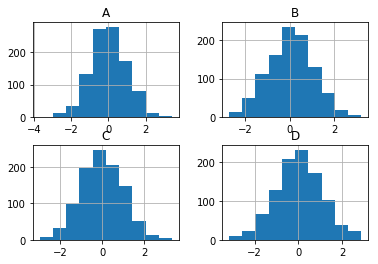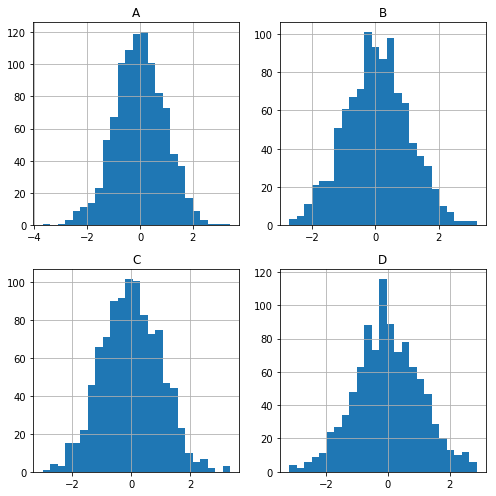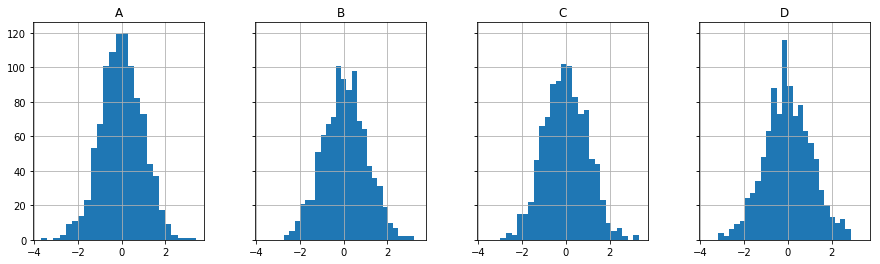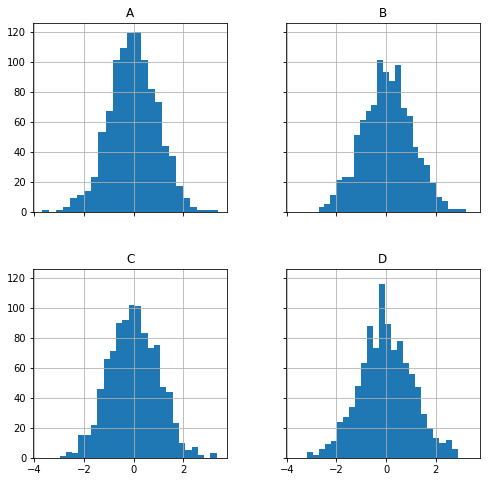# pandas_data_viz_1

## Data visualization with Pandas¶¶

Pandas implements some high level plotting functions using matplotlib. Note If you have seaborn imported, pandas will relay the plotting through seaborn and you get better looking plots for the same data and commands.

In :
```import pandas as pd
import numpy as np
%matplotlib inline
import matplotlib.pyplot as plt
import seaborn as sns
```

In :
```df1 = pd.read_csv('/Users/atma6951/Documents/code/pychakras/pychakras/udemy_ml_bootcamp/Python-for-Data-Visualization/Pandas Built-in Data Viz/df1', index_col=0)
df2 = pd.read_csv('/Users/atma6951/Documents/code/pychakras/pychakras/udemy_ml_bootcamp/Python-for-Data-Visualization/Pandas Built-in Data Viz/df2')
```
In :
```df1.head()
```
Out:
A B C D
2000-01-01 1.339091 -0.163643 -0.646443 1.041233
2000-01-02 -0.774984 0.137034 -0.882716 -2.253382
2000-01-03 -0.921037 -0.482943 -0.417100 0.478638
2000-01-04 -1.738808 -0.072973 0.056517 0.015085
2000-01-05 -0.905980 1.778576 0.381918 0.291436
In :
```df2.head()
```
Out:
a b c d
0 0.039762 0.218517 0.103423 0.957904
1 0.937288 0.041567 0.899125 0.977680
2 0.780504 0.008948 0.557808 0.797510
3 0.672717 0.247870 0.264071 0.444358
4 0.053829 0.520124 0.552264 0.190008

### 3 ways of calling plot from a DataFrame¶¶

• `df.plot()` and specify the plot type, the X and Y columns etc
• `df.plot.hist()` calling plot in OO fashion. Only specify teh X and Y and color or size columns
• `df['column'].plot.plotname()` - calling plot on a series

Types of plot that can be called: area, bar, line, scatter, box, hexbin, kde etc.

#### Ways of plotting histogram¶¶

In :
```df1.plot(x='A', kind='hist')
```
Out:
`<matplotlib.axes._subplots.AxesSubplot at 0x1142ece80>`In :
```df1['A'].plot.hist(bins=30)
```
Out:
`<matplotlib.axes._subplots.AxesSubplot at 0x1169717f0>`#### Plotting a histogram of all numeric columns in the dataframe:¶¶

In :
```df1.hist()
```
Out:
```array([[<matplotlib.axes._subplots.AxesSubplot object at 0x11a872eb8>,
<matplotlib.axes._subplots.AxesSubplot object at 0x11abd27f0>],
[<matplotlib.axes._subplots.AxesSubplot object at 0x11abf7e80>,
<matplotlib.axes._subplots.AxesSubplot object at 0x11ac27550>]],
dtype=object)```In reality, you have a lot more columns. You can prettify the above by creating a layout and figsize:

In :
```ax_list = df1.hist(bins=25, layout=(2,2), figsize=(7,7))
plt.tight_layout()
```#### Plotting histogram of all columns and sharing axes¶¶

The chart above might make more sense if you shared the X as well as Y axes for different columns. This helps in comparing the distribution of values visually.

In :
```ax_list = df1.hist(bins=25, sharex=True, sharey=True, layout=(1,4), figsize=(15,4))
```In :
```ax_list = df1.hist(bins=25, sharex=True, sharey=True, layout=(2,2), figsize=(8,8))
```### Backgrounds¶¶

You can specify dark or white background and style info to the matplotlib that is used behind the scenes.

#### Area plot¶¶

In :
```plt.style.use('dark_background')
df2.plot.area()
```
Out:
`<matplotlib.axes._subplots.AxesSubplot at 0x116b35f98>`#### Bar chart¶¶

Another style is `fivethirtyeight`

In :
```plt.style.use('fivethirtyeight')
df2.plot.bar()
```
Out:
`<matplotlib.axes._subplots.AxesSubplot at 0x116c92cc0>`#### Line plot¶¶

This is suited for time series data

In :
```#reset the style
plt.style.use('default')

# pass figsize to the matplotlib backend engine and `lw` is line width
df1.plot.line(x=df1.index, y='A', figsize=(12,2), lw=1)
```
Out:
`<matplotlib.axes._subplots.AxesSubplot at 0x116f61f98>`#### Scatter plot¶¶

Use `colormap` or `size` to bring in a visualize a 3rd variable in your scatter

In :
```df1.plot.scatter(x='A', y='B',c='C', cmap='coolwarm')
```
Out:
`<matplotlib.axes._subplots.AxesSubplot at 0x1176e09b0>`In :
```# you could specify size s='c' however the points come out tiny.
# had to scale it by 100, hence using actual series data and not the column name
df2.plot.scatter(x='a',y='b', s=df2['c']*100)
```
Out:
`<matplotlib.axes._subplots.AxesSubplot at 0x117440f60>`#### KDE plots¶¶

To visualize the density of data

In :
```df1['A'].plot.kde()
```
Out:
`<matplotlib.axes._subplots.AxesSubplot at 0x117a9d860>`Visualize the density of all columns in one plot

In :
```df1.plot.kde()
```
Out:
`<matplotlib.axes._subplots.AxesSubplot at 0x117d5e048>`In :
```df2.plot.density() #I think density is an alias to KDE
```
Out:
`<matplotlib.axes._subplots.AxesSubplot at 0x1180b45f8>`### Making wordclouds from text fields¶¶

Word clouds are a great way to visualize frequency of certain terms that appear in the data set. This is accomplished using the library wordcloud. You can install it as

``conda install -c conda-forge wordcloud``
In :
```registrant_df = pd.read_csv('./registrant.csv')
```
Out:
Unnamed: 0 Registration Date Country Organization Current customer? What would you like to learn?
0 0 11/08/2019 06:09 PM EST Jamaica The University of the West Indies NaN NaN
1 1 11/08/2019 06:09 PM EST Japan iLand6 Co.,Ltd. no I am interested ArcGIS.
2 2 11/08/2019 05:56 PM EST Canada Safe Software Inc yes data science workflos
3 3 11/08/2019 05:51 PM EST Canada Le Groupe GeoInfo Inc yes general information
4 4 11/08/2019 05:26 PM EST Canada Safe Software Inc. NaN NaN

Now, let us plot the responses from the column `What would you like to learn?` as a word cloud. First, we need to turn the series into a paragraph.

In :
```obj_series = registrant_df['What would you like to learn?'].dropna()
obj_list = list(obj_series)
obj_string = ' '.join(obj_list)
obj_string
```
Out:
`"I am interested ArcGIS. data science workflos general information integration of arcgis and jupyter notebooks Using tools like pandas to perform ETL functions on GIS data; reading/writing to/from spatial data frames to GIS formats quickly working with Jupyter notebooks More use cases for Jupytr notebooks in ArcGIS Datascience and GIS Integration, ArcGIS Deep learning concepts ands evaluation How to carefully clean and prepare data for statistical computations The jupyter integration better use of the web environment via Jupyter, including how to share with colleagues, etc Notebook stuff All about ESRI: Modellization, Land use land cover mapping, mapping land degradation, mapping soil erosion using this for community projects Jupyter Integration of Jupyter Notebooks to workflows all the things Anything Deep Learning with Remote Sensing use python Improved humanitarian data collection, analysis and visualization Process Flow The basics How to use Jupyter and ArcGIS notebooks to facilitate efficient workflows Jupiter's analytical capability Methods for automating workflows, increasing accuracy and documenting methods Geoanalytics How to use ArcGIS and Jupyter for Geospatial science data analysis More basics about Jupyter and the Esri Notebooks tool too. Workflow to clean, analyse and visualize data from start to finish how to discuss with executives and professors to use this collaboration platform How to use Jupyter and ArcGIS API for Python Any thing new. open source Python libraries . Notebook Data Science for Geospatial Analysis How we can best use Jupyter in our existing and future solutions How to use Jupyter notebook. More details about Jupyter using python with arcgis Learn about using Jupyter notebooks in spatial analysis How to link between ArcGIS and Jupyter Geospatial analysis using python More about how to use Jupiter Notebooks. Jupyter notebooks a bit better colleague mail python scripting Use of Jupyter Potential for analysis workflows notebook, jupyter Use of Jupyter Notebooks stuff Better ways to integrate Python into engineering workflows throughout our company whats new About Jupyter How to setup arcgis and jupyter notebooks Setting up Jupiter notebooks / environments management install and environment set up, licensing Python Programming, Data Science, Machine Learning Data Science tools in ArcPy How to combine ArcGIS Tools and Jupyter Data Science techniques things to make my job more efficient Please provide examples with data items that don't expires (like the majority of those on your developer examples website) Data interoperability opportunities and constraints between ESRI's proprietary data formats and Python open source standards In general, more about data science The integration between the two platforms What is and how to use ArcGIS Notebooks and Jupyter Use case to notebooks as developer How to Use ArcGIS and Jupyter use Jupyter to increase transparency and build reproducible research one of the topics I have been lately exploring is how to make the maps web-enabled? More about Jupyter Notebooks I'm familiar with Jupyter but I'd like to know best practices Geospatial data science Geospatial Datascienc How Jupyter can help my organization. the things i don't know that i don't know. awareness How to use the Jupyter Notebook environment to perform analysis that's not available in ArcGIS Pro because of licensing. Data Science and use of python Looking to improve my data analysis capabilities and how I can integrate into ArcGIS more about Juypter notebooks and how it can fit into ESRI workflows How to use the Jupyter notebooks version of ESRI and what do I need to get started within my company... tools for extracting centreline from real time point data(i.e. cell phone locations, AIS locations) I would like to see if these workflows would be applicable to my job. general knowledge What notebooks and Jupyter can do for disaster response. Data analytics Content as advertised How to apply ArcGIS Notebooks DATA SCIENCE WITH GIS Geospatial data science workflow analysis Current trends in data science I would like to learn both how I can improve my GIS skills and how to use other software relevant to GIS and mapping. More about notebooks and GIS All related to this topics Jupyter usage General knowledge About Notebooks about the topic How to implement ArcGIS notebooks at an enterrpise scale. What it says! initiation to data science applied to geomatics exmples of application of ArcGIS with Jupyter data science, jupyter noteboook"`
In :
```from wordcloud import WordCloud
wc = WordCloud(width=1000, height=600, background_color='white')
```
In :
```obj_wc_img = wc.generate_from_text(obj_string)
```
In :
```plt.figure(figsize=(20,10))
plt.imshow(obj_wc_img, interpolation="bilinear")
plt.axis('off')
plt.title('What would you like to learn?');
```